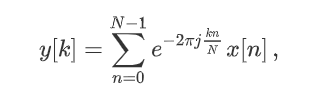Skip to content
Related Articles
scipy.fft() in Python
• Last Updated : 29 Aug, 2020

With the help of scipy.fft() method, we can compute the fast fourier transformation by passing simple 1-D numpy array and it will return the transformed array by using this method.Fast Fourier Transformation

Syntax : scipy.fft(x)

Return : Return the transformed array.

Example #1 :

In this example we can see that by using scipy.fft() method, we are able to compute the fast fourier transformation by passing sequence of numbers and return the transformed array.

## Python3

 `# import scipy and numpy``import` `scipy``import` `numpy as np`` ` `x ``=` `np.array(np.arange(``10``))``# Using scipy.fft() method``gfg ``=` `scipy.fft(x)`` ` `print``(gfg)`

Output :

[45. +0.j         -5.+15.38841769j -5. +6.8819096j  -5. +3.63271264j

-5. +1.62459848j -5. +0.j         -5. -1.62459848j -5. -3.63271264j

-5. -6.8819096j  -5.-15.38841769j]

Example #2 :

## Python3

 `# import scipy and numpy``import` `scipy``import` `numpy as np`` ` `x ``=` `np.array(np.arange(``5``))``# Using scipy.fft() method``gfg ``=` `scipy.fft(x)`` ` `print``(gfg)`

Output :

[10. +0.j         -2.5+3.4409548j  -2.5+0.81229924j -2.5-0.81229924j

-2.5-3.4409548j ]

Attention geek! Strengthen your foundations with the Python Programming Foundation Course and learn the basics.

To begin with, your interview preparations Enhance your Data Structures concepts with the Python DS Course. And to begin with your Machine Learning Journey, join the Machine Learning – Basic Level Course

My Personal Notes arrow_drop_up